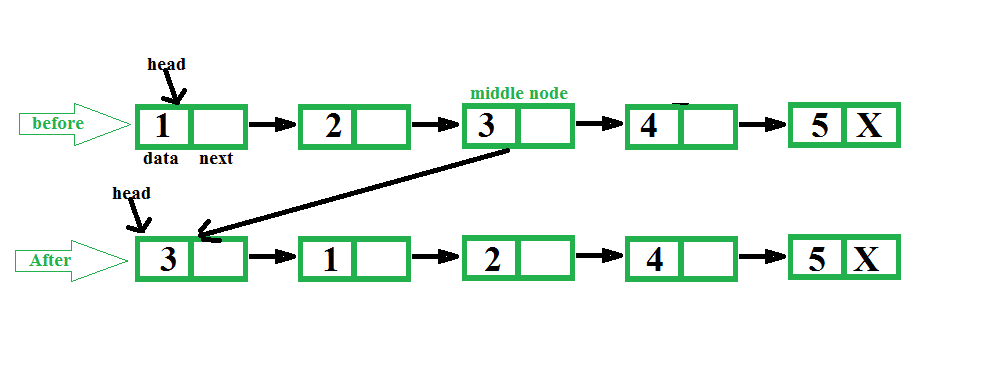GeeksforGeeks App
Open AppBrowser
Continue

# Python Program For Making Middle Node Head In A Linked List

Given a singly linked list, find middle of the linked list and set middle node of the linked list at beginning of the linked list.
Examples:

```Input: 1 2 3 4 5
Output: 3 1 2 4 5

Input: 1 2 3 4 5 6
Output: 4 1 2 3 5 6 ```The idea is to first find middle of a linked list using two pointers, first one moves one at a time and second one moves two at a time. When second pointer reaches end, first reaches middle. We also keep track of previous of first pointer so that we can remove middle node from its current position and can make it head.

## Python3

 `# Python3 program to make middle node``# as head of Linked list` `# Linked List node``class` `Node:``    ``def` `__init__(``self``, data):``        ``self``.data ``=` `data``        ``self``.``next` `=` `None` `# function to get the middle node``# set it as the beginning of the``# linked list``def` `setMiddleHead(head):``    ``if``(head ``=``=` `None``):``        ``return` `None` `    ``# To traverse nodes``    ``# one by one``    ``one_node ``=` `head` `    ``# To traverse nodes by``    ``# skipping one``    ``two_node ``=` `head` `    ``# To keep track of previous middle``    ``prev ``=` `None` `    ``while``(two_node !``=` `None` `and``          ``two_node.``next` `!``=` `None``):` `        ``# For previous node of middle node``        ``prev ``=` `one_node` `        ``# Move one node each time``        ``one_node ``=` `one_node.``next` `        ``# Move two nodes each time``        ``two_node ``=` `two_node.``next``.``next` `    ``# Set middle node at head``    ``prev.``next` `=` `prev.``next``.``next``    ``one_node.``next` `=` `head``    ``head ``=` `one_node` `    ``# Return the modified head``    ``return` `head` `def` `push(head, new_data):` `    ``# Allocate new node``    ``new_node ``=` `Node(new_data)` `    ``#Link the old list to new node``    ``new_node.``next` `=` `head` `    ``# Move the head to point the new node``    ``head ``=` `new_node` `    ``# Return the modified head``    ``return` `head` `# A function to print a given linked list``def` `printList(head):``    ``temp ``=` `head``    ``while` `(temp!``=``None``):``        ` `        ``print``(``str``(temp.data), end ``=` `" "``)``        ``temp ``=` `temp.``next``    ``print``("")` `# Create a list of 5 nodes``head ``=` `None``for` `i ``in` `range``(``5``, ``0``, ``-``1``):``    ``head ``=` `push(head, i)` `print``(``" list before: "``, end ``=` `"")``printList(head)` `head ``=` `setMiddleHead(head)` `print``(``" list After: "``, end ``=` `"")``printList(head)``# This code is contributed by Pranav Devarakonda`

Output:

```list before: 1 2 3 4 5
list After : 3 1 2 4 5 ```

Time complexity: O(n) where n is the size of the linked list

Space Complexity: O(1) since using constant space